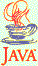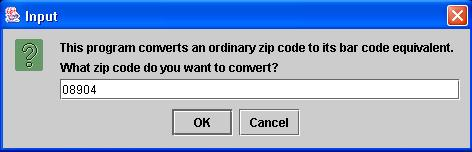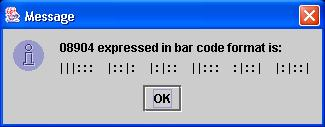### Bar Codes

20 points

The post office uses a scheme for converting an ordinary zip code, such as 08904, into a bar code. In this program you do the conversion. For example, here is the box that pops up when my program starts:After the program does its calculations, this box pops up with the result:Here is how the post office's conversion scheme works. Each digit of a zip code is converted into a 5-digit binary number. For example, a '1' in a zip code is always converted into '00011'. Then the binary number is converted into a bar code. The 0's in the number are displayed as short bars (:), and the 1's as tall bars (|). The bar code at this point has 5 x 5 = 25 bars. An example is shown on p. 327 in the text.

A 6th digit, called a 'correction digit', is also displayed in the bar code. To find the correction digit, first add together the 5 digits of the ordinary zip code. For 08904, the sum is 0 + 8 + 9 + 0 + 4 = 21. The correction digit is the number you'd have to add to this sum to reach a multiple of 10. The nearest multiple of 10 is 30, so the correction digit here is 9. This 9 would be converted to a 5-digit binary number, and then to short and tall bars, and would be displayed as the 6th digit in the bar code. The bar code at this point has 6 x 5 = 30 bars.

Finally, to complete the bar code, 1 frame bar (|) is added both before and after the number. The final bar code has 32 bars.

Here is the table that the post office uses to convert the digits 0 to 9 into 5-digit binary numbers:

 Digit Binary Number 0 11000 1 00011 2 00101 3 00110 4 01001 5 01010 6 01100 7 10001 8 10010 9 10100

Use classes Digit, BarCode and BarCodeVisualizer in your solution. In each class, write methods to do specific tasks. Here is how I broke down the various tasks (but you can set it up differently):

Digit
This class handles tasks related to converting a 5-digit zip code into a string of 0's and 1's.

• A method that converts a single digit of an ordinary zip code into its associated 5-digit binary number
• A method that finds the correction digit, the 6th digit in the bar code
• A method that converts the entire 5-digit zip code into a 32-character string of 0's and 1's. This method will call both methods listed above. It should add a '1' both before and after the 30-character (6 x 5) binary number for the 'frame bars'.

BarCode
This class converts a string of 0's and 1's into a bar code.

• A method that first makes a call to 'new' to create an object of type 'BarCodeVisualizer.' Then it steps through the 32 characters of the binary-form zip code and creates a (bar code) string with short and tall bars. If a given character is '0' the method calls the 'addShortBar(String)' method of the 'BarCodeVisualizer' object. If a character is '1' the method calls the 'addTallBar(String)' method of 'BarCodeVisualizer'. The method then calls the 'addSpace(String)' method of 'BarCodeVisualizer' if it is the 5th, 10th, 15th, 20th or 25th character in the binary-form zip code -- to put some space after each of the first 5 digits of the bar code.

BarCodeVisualizer
This class handles the visual aspects of the bar code -- how does the bar code look when it is displayed? It is generally a good idea in object-oriented design to separate the visual, display aspects of a program from its other operations, and this class does that here.

• A method 'addShortBar(String s)' that appends ': ' to 's' and then returns it.
• A method 'addTallBar(String s)' that appends '| ' to 's' and returns it.
• A method 'addSpace(String s)' that appends several spaces to 's' and returns it.

main()
You can put 'main' either in one of these 3 classes or in a separate 4th class called 'BarCodeGenerator', which has only 'main' in it. (I wrote a separate class 'myBarCodeGenerator.java' to hold 'main()'.) In 'main', ask the user for a zip code. Create a Digit object, and send the zip code to the Digit object for processing. Create a BarCode object, take the result from the Digit object, and send it to the BarCode object for further work. Finally, display the bar code that the BarCode object returns to 'main'.

Although this program deals with numbers, I found it convenient to use mostly Strings in storing and working with the zip codes. Use the 'substring()' method of the String class to extract small strings from larger strings. For example, 'myString.substring(i, i+1)' gives you the 'ith' character in 'myString'.

Use 'JOptionPane.showInputDialog()' to get input and 'JOptionPane.showMessageDialog(null, String x)' to display output. Import 'javax.swing.JOptionPane'.

### Getting Started

You can download BarCode.zip which contains the 4 interacting classes that you need to write for this program.

### Credit

This programming assignment is based directly on Exercise P7.11 in Computing Concepts with Java Essentials, Third Edition, by Cay Horstmann.